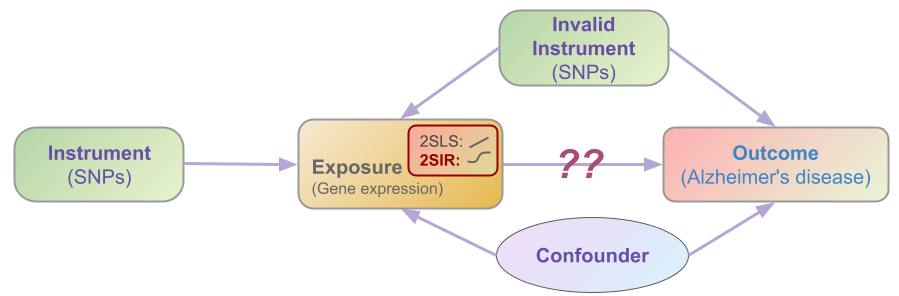# 🧬 nonlinear-causal#nonlinear-causal is a Python module for nonlinear causal inference, including hypothesis testing and confidence interval for causal effect, built on top of two-stage methods.

The proposed model is:$\text{(Stage 1)} \quad \phi(x) = \mathbf{z}^\prime \boldsymbol{\theta} + w, \qquad \text{(Stage 2)} \quad y = \beta \phi(x) + \mathbf{z}^\prime \boldsymbol{\alpha} + \epsilon$

## 🎯 What We Can Do#

• Estimate marginal causal effect $$\beta$$

• Hypothesis testing (HT) and confidence interval (CI) for marginal causal effect $$\beta$$.

• Estimate nonlinear causal link $$\phi(\cdot)$$.

## #️⃣ Reference#

If you use this code please star 🌟 the repository and cite the following paper:

• Dai, B., Li, C., Xue, H., Pan, W., & Shen, X. (2022). Inference of nonlinear causal effects with GWAS summary data. arXiv preprint arXiv:2209.08889.

@article{dai2022inference,
title={Inference of nonlinear causal effects with GWAS summary data},
author={Dai, Ben and Li, Chunlin and Xue, Haoran and Pan, Wei and Shen, Xiaotong},
journal={arXiv preprint arXiv:2209.08889},
year={2022}
}